## Percentage For SBI PO : Set – 12

D.1-8) What should come in place of questions mark (?) in the following questions?

1) 85% of ? = 3060

a) 3300

b) 3600

c) 4200

d) 4800

e) None of these

b)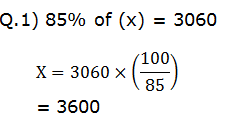2) ?% of 240 = 43.2

a) 14

b) 16

c) 18

d) 20

e) 24

c)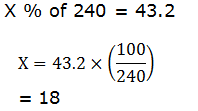3) ?% of 924 + 39 = 371.64

a) 31

b) 33

c) 35

d) 37

e) None of these

e)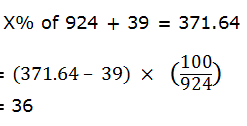4) 55% of ? – 72 = 1110.5

a) 2095

b) 2140

c) 2150

d) 2195

e) None of these

c)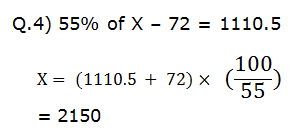5) (0.05% of 364 + 0.2% of 152) × 400 = ?

a) 136.8

b) 152.5

c) 175.6

d) 194.4

e) 199.2

d)

(0.05% of 364 + 0.2% of 152) × 400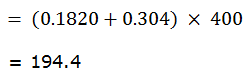6) (0.45% of 150) × (3.25% of 240) = ?

a) 4.135

b) 5.265

c) 6.185

d) 9.715

e) none of these

b)

(0.45% of 150) × (3.25% of 240)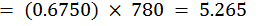7) (6.5% of 130) – (0.24% of 750) = ? % of 13.3

a) 50

b) 65

c) 90

d) 105

e) 120

a)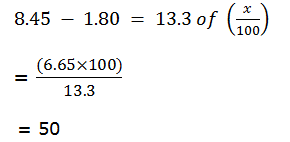8) 1.25 × 1.5 + 4% of 160 = ?

a) 18.186

b) 15.245

c) 12.962

d) 8.275

e) None of these

d)

1.25 × 1.5 + 4% of 160

= 1.875 + 6.4

=8.275

9)  In a company XYZ, the respective ratio between the total number of under-graduate employees and the total number of post-graduate employees is 13 : 23. The company hasonly two branches, one in Mumbai and one in Delhi. If the total number of undergraduate employees in Mumbai branch is 351, which is 30% of the total undergraduate employees in the company, what is the total number of post-graduateemployee in the company ?

a) 2185

b) 1955

c) 2070

d) 2100

e) 1985

c)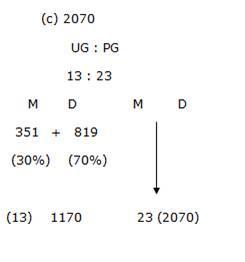10) Akash scored 73 marks in subject A. He scored 56% marks in subject B and X marks in subject C. Maximum marks in each subject were 150.The overall percentage marks obtained by Akash in all the three subjects together were 54%. How many marks did he score in subject C ?

a) 84

b) 86

c) 79

d) 73

e) None of these

b)

Mark = 73

Maxi mark = 150

A scored = 56%-> B and x marks in subject C

How many mark did he score in sub C?

C =(73+84+x/450)*100=54

X = 86